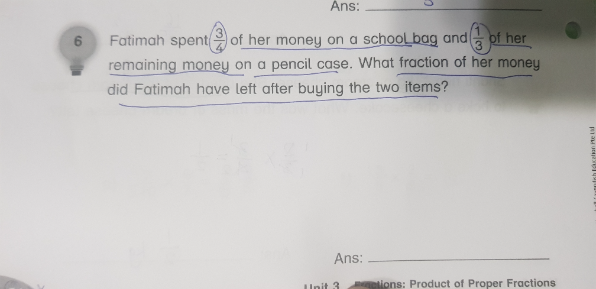# Question```Method 1:
Let M be money at the start.
Spent 3/4 M on school bag, so remaining is 1/4 M.
Spent 1/3 of 1/4 M on pencil case, so left 2/3 x 1/4 M = 1/6 M.
So she has 1/6 of money left.

Method 2:
Let 12u be money at the start. (denominators 3 and 4 suggests 3x4=12 units)
School bag is 3/4 x 12u = 9u. So left 3u.
Pencil case is 1/3 x 3u = u. So left 2u.
Amount left is 2u/12u = 1/6 of money at the start.

```
0 Replies 2 Likes ✔Accepted Answer

Let u be the total units of money.

Spent  School bag         ( 3/4) u

Remaining \$                   (1/4)u

Spent Pencil case          (1/3)(1/4)u = (1/12)u

Money left = u(3/4)u(1/12)u = (12/12) u -(9/12)u – (1/12) u = (2/12) u = (1/6)u

Hence fraction of money left = 1/6

0 Replies 2 Likes